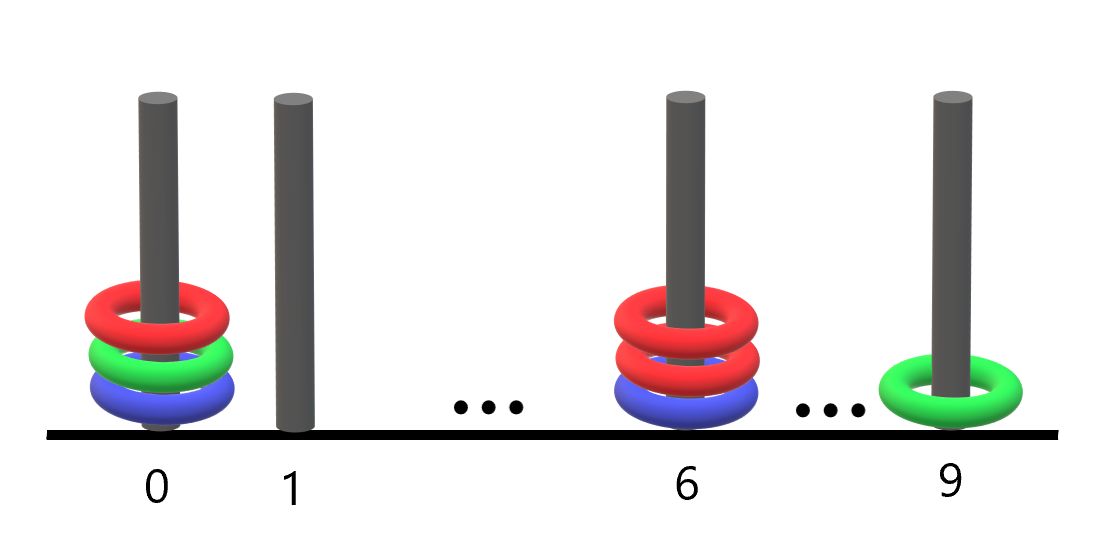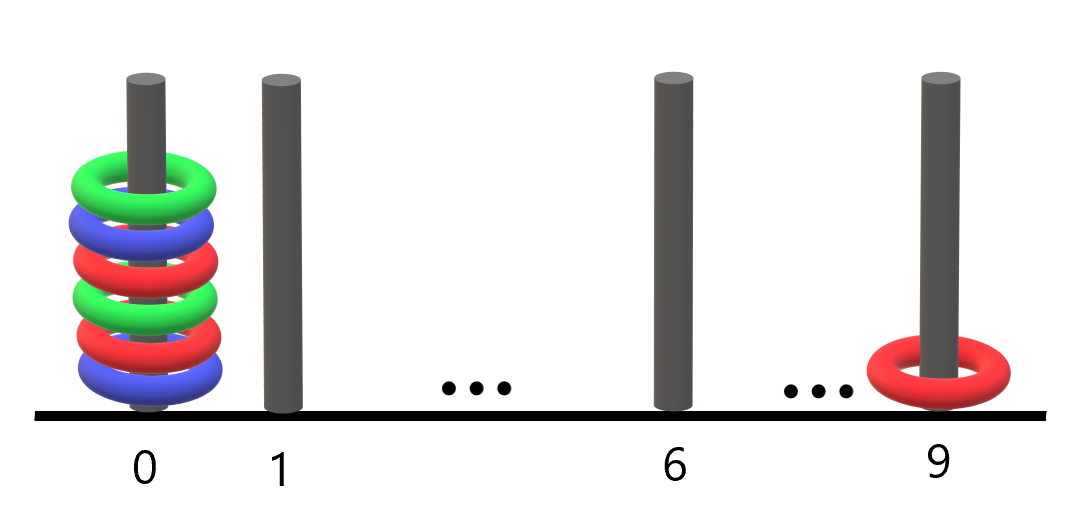##### Welcome to Subscribe On Youtube

Formatted question description: https://leetcode.ca/all/2103.html

# 2103. Rings and Rods (Easy)

There are n rings and each ring is either red, green, or blue. The rings are distributed across ten rods labeled from 0 to 9.

You are given a string rings of length 2n that describes the n rings that are placed onto the rods. Every two characters in rings forms a color-position pair that is used to describe each ring where:

• The first character of the ith pair denotes the ith ring's color ('R', 'G', 'B').
• The second character of the ith pair denotes the rod that the ith ring is placed on ('0' to '9').

For example, "R3G2B1" describes n == 3 rings: a red ring placed onto the rod labeled 3, a green ring placed onto the rod labeled 2, and a blue ring placed onto the rod labeled 1.

Return the number of rods that have all three colors of rings on them.

Example 1:Input: rings = "B0B6G0R6R0R6G9"
Output: 1
Explanation:
- The rod labeled 0 holds 3 rings with all colors: red, green, and blue.
- The rod labeled 6 holds 3 rings, but it only has red and blue.
- The rod labeled 9 holds only a green ring.
Thus, the number of rods with all three colors is 1.


Example 2:Input: rings = "B0R0G0R9R0B0G0"
Output: 1
Explanation:
- The rod labeled 0 holds 6 rings with all colors: red, green, and blue.
- The rod labeled 9 holds only a red ring.
Thus, the number of rods with all three colors is 1.


Example 3:

Input: rings = "G4"
Output: 0
Explanation:
Only one ring is given. Thus, no rods have all three colors.


Constraints:

• rings.length == 2 * n
• 1 <= n <= 100
• rings[i] where i is even is either 'R', 'G', or 'B' (0-indexed).
• rings[i] where i is odd is a digit from '0' to '9' (0-indexed).

Similar Questions:

## Solution 1. Counting

// OJ: https://leetcode.com/problems/rings-and-rods/
// Time: O(N)
// Space: O(1)
class Solution {
public:
int countPoints(string s) {
bool cnt = {};
for (int i = 0; i < s.size(); i += 2) {
int color = s[i] == 'R' ? 0 : (s[i] == 'G' ? 1 : 2), index = s[i + 1] - '0';
cnt[index][color] = true;
}
int ans = 0;
for (int i = 0; i < 10; ++i) {
ans += cnt[i] && cnt[i] && cnt[i];
}
return ans;
}
};


## Solution 2. Counting with Bitmask

// OJ: https://leetcode.com/problems/rings-and-rods/
// Time: O(N)
// Space: O(1)
class Solution {
public:
int countPoints(string s) {
char cnt = {};
for (int i = 0; i < s.size(); i += 2) {
int color = s[i] == 'R' ? 0 : (s[i] == 'G' ? 1 : 2), index = s[i + 1] - '0';
cnt[index] |= 1 << color;
}
int ans = 0;
for (int i = 0; i < 10; ++i) ans += cnt[i] == 0b111;
return ans;
}
};